# Poly-harmonic function

hyper-harmonic function, meta-harmonic function, of orderA functionof real variables defined in a regionof a Euclidean space,, having continuous partial derivatives up to and including the orderand satisfying the poly-harmonic equation everywhere in:whereis the Laplace operator. For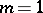one obtains harmonic functions (cf. Harmonic function), while for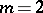one obtains biharmonic functions (cf. Biharmonic function). Each poly-harmonic function is an analytic function of the coordinates. Some other properties of harmonic functions also carry over, with corresponding changes, to poly-harmonic functions.

For poly-harmonic functions of any order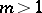, representations using harmonic functions are generalized to get results known for biharmonic functions . For example, for a poly-harmonic functionof two variables there is the representationwhere,, are harmonic functions in. For a functionof two variables to be a poly-harmonic function, it is necessary and sufficient that it be the real (or imaginary) part of a poly-analytic function.

The basic boundary value problem for a poly-harmonic function of orderis as follows: Find a poly-harmonic function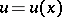in a regionthat is continuous along with its derivatives up to and including the order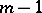in the closed regionand which satisfies the following conditions on the boundary: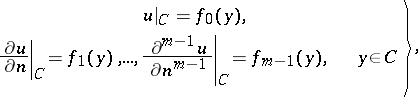(*)

whereis the derivative along the normal toandare given sufficiently smooth functions on the sufficiently smooth boundary. Many studies deal with solving problem (*) in the ball in, . To solve the problem (*) in the case of an arbitrary region, one uses methods of integral equations, as well as variational methods , .